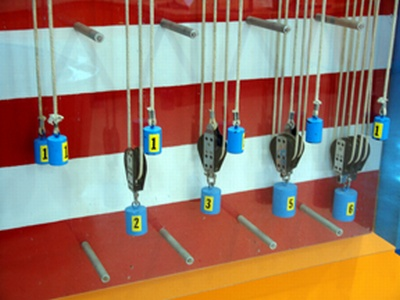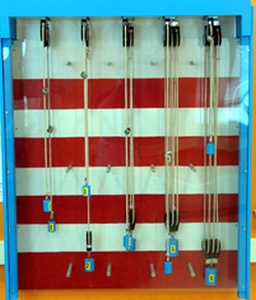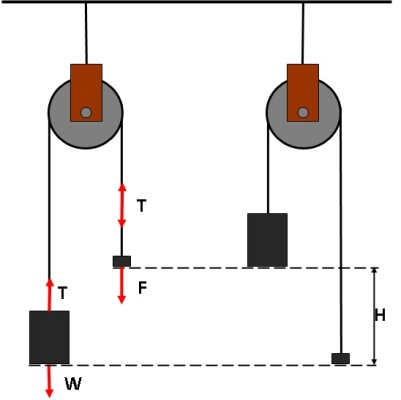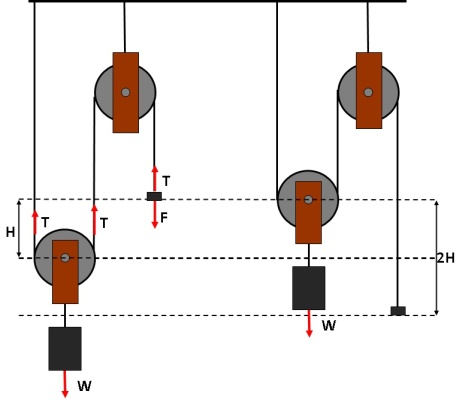# PulleysPulleys are mechanisms compost by wheel and rope used to lift heavy objects onto tall heights. They change the direction of an applied force and they can even reduce the force needed to lift a weight. Pulleys systems are common used in constructions.

In the exhibit, systems of one to five pulleys are uses to lift a weight of 1lb onto a 1 ft height.

## A SIMPLE BLOCK AND TACKLE

In order to lift the 1lb weight W you have to apply a force of F on the rope equal to the weight W. The rope is now under a tension T equal to the force F.

To lift this weight a distance of H=1 ft you will have to pull in a length L= H=1 ft of the rope.

The mechanical advantage M is one: M=W/F=1.In order to lift the 1lb weight W you have to apply a force of  F  on the rope. Like in the simple case of the block and tackle the rope is under a tension T equal to the applied force F. But in this case the weight is supported by twice the tension: 2T=W. The force F you have to apply then is only half of the weight W.

By having the second pulley you have decreased the force needed to lift the weight. The mechanical advantage M is now two: M=W/F=2T/T=2.

However, order to lift the weight a distance of   H=1 ft you will have to pull in a length L = H x M =2 ft of the rope.

You gained by having to apply a smaller force, but had to compensate by having to pull a longer length of rope.